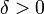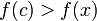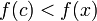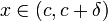# First derivative test

## Statement

### What the test is for

The first derivative test is a partial (i.e., not always conclusive) test used to determine whether a particular critical point in the domain of a function is a point where the function attains a local maximum value, local minimum value, or neither. There are cases where the test is inconclusive, which means that we cannot draw any conclusion.

### What the test says: one-sided sign versions

Suppose$f$ is a function defined around a point$c$ (i.e.,$f$ is defined in an open interval containing$c$) and is continuous at$c$ (What this means is that we do not care whether$f$ is differentiable at$c$; however, it must be continuous at$c$ and differentiable at points to the immediate left and immediate right of$c$).

Then, we have the following:

Hypothesis Conclusion$\! f'(x)$ is positive (respectively, nonnegative) for$x$ to the immediate left of$c$ (i.e., for$x \in (c - \delta, c)$ for sufficiently small$\delta > 0$)$f$ has a strict local maximum from the left at$c$, i.e.,$f(c) > f(x)$ (respectively,$f$ has a local maximum from the left at$c$, i.e.,$f(c) \ge f(x)$) for$x$ to the immediate left of$c$.$\! f'(x)$ is negative (respectively, nonpositive) for$x$ to the immediate left of$c$ (i.e., for$x \in (c - \delta, c)$ for sufficiently small$\delta > 0$)$f$ has a strict local minimum from the left at$c$, i.e.,$f(c) < f(x)$ (respectively,$f$ has a local minimum from the left at$c$, i.e.,$f(c) \le f(x)$) for$x$ to the immediate left of$c$.$\! f'(x)$ is positive (respectively, nonnegative) for$x$ to the immediate right of$c$ (i.e., for$x \in (c,c + \delta)$ for sufficiently small$\delta > 0$)$f$ has a strict local minimum from the right at$c$, i.e.,$f(c) < f(x)$ (respectively,$f$ has a local minimum from the right at$c$, i.e.,$f(c) \le f(x)$) for$x$ to the immediate right of$c$.$\! f'(x)$ is negative (respectively, nonpositive) for$x$ to the immediate right of$c$ (i.e., for$x \in (c,c + \delta)$ for sufficiently small$\delta > 0$)$f$ has a strict local maximum from the right at$c$, i.e.,$f(c) > f(x)$ (respectively,$f$ has a local maximum from the right at$c$, i.e.,$f(c) \ge f(x)$) for$x$ to the immediate right of$c$.

### What the test says: combined sign versions

Suppose$f$ is a function defined around a point$c$ (i.e.,$f$ is defined in an open interval containing$c$) and is continuous at$c$ (What this means is that we do not care whether$f$ is differentiable at$c$; however, it must be continuous at$c$ and differentiable at points to the immediate left and immediate right of$c$).

Then, we have the following (we list only the strict cases in the table below):

Sign of$f'$ on immediate left of$c$ Sign of$f'$ on immediate right of$c$ Conclusion about local minimum, local maximum, or neither
positive negative strict local maximum
positive positive neither local maximum nor local minimum
negative negative neither local maximum nor local minimum
negative positive strict local minimum

If we replace positive by nonnegative and negative by nonpositive in the rows corresponding to strict local maximum and strict local minimum, we could potentially lose the strictness.

### Succinct version

Here is a shorter version: at a critical point, if the derivative changes sign from negative to positive (as we go from left to right) then that is a point of local minimum. If the derivative changes sign from positive to negative (as we go from left to right) then it is a point of local maximum.